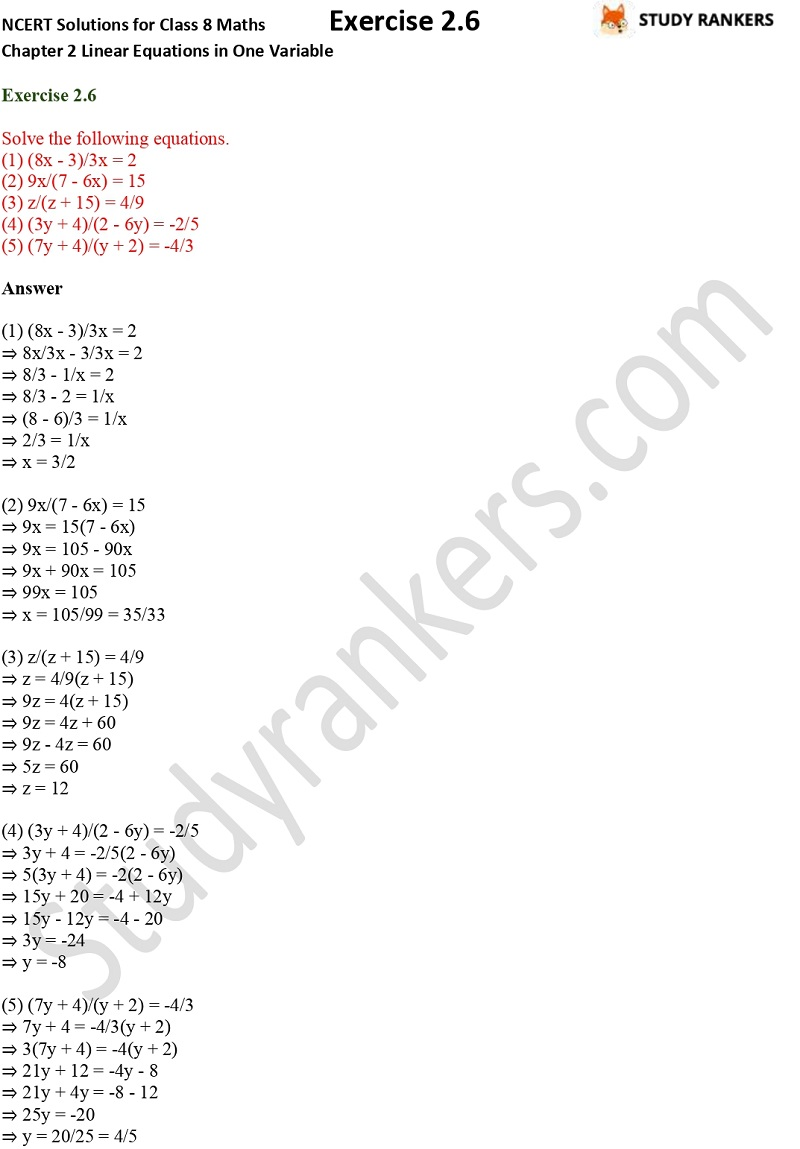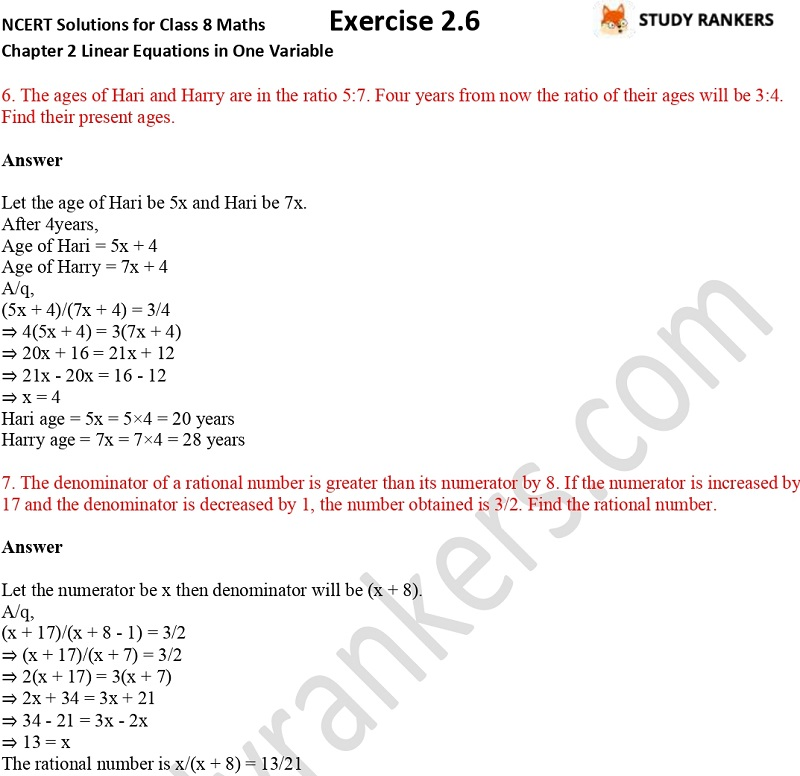>

## NCERT Solutions for Class 8 Maths Chapter 2 Linear Equations in One Variable Exercise 2.6

If you're facing trouble in finding the correct NCERT Solutions of Chapter 2 Linear Equations in One Variable Exercise 2.6 Class 8 Maths then you can find them here. We have provided detailed NCERT Solutions for Class 8 Maths which will help you in improving problem solving skills. These NCERT questions and answers are prepared by Studyrankers which are updated as per the latest syllabus of CBSE. You can take help of these if you ever got stuck while solving any question and score good marks in the examinations.

Exercise 2.6 has only two questions which are about solving given equations and word problems.